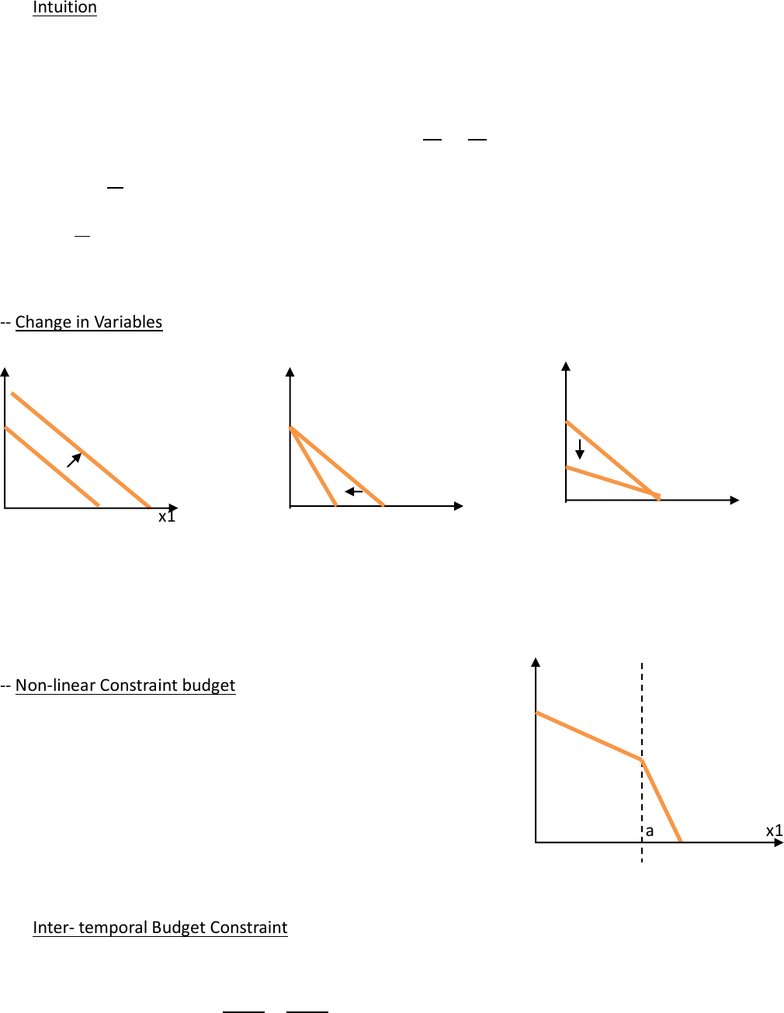Class Notes (1,100,000)
CA (630,000)
UTSG (50,000)
ECO (2,000)
Lecture

# Topic 3 - Budget Constraint Budget Constraint.

Department
Economics
Course Code
ECO206Y1
Professor
Loren Brandt

Page:
of 2Topic 3 - Budget Constraints
(Lecture 2 Sept 21st)
Intuition:
1. Goods: x1, x2;
2. Price: p1, p2
3. E: total expenditure/budget the individual has;
Assume that all of E is spent, E = p1x1 + p2x2 


Y-intercepts:
 = maximum units of x2 that can be consumed;
Slope: 
 = OC = Units of x2 that have to be reduced to consume one more unit of x1;
-- Change in Variables:
x2 x2 x2
x1 x1 x1
E shifts outward p1 steeper p2 flatter
-- Non-linear Constraint budget x2
Liner budget constraint = assumption that prices are fixed;
In the real world, there might be non-linearity of prices
e.g. price of electricity depends on usage
x1<a, p1;
x1>a,2p1;
x2,p2 a x1
Inter- temporal Budget Constraint
Assume Y1,Y2 “income” in two periods;
x1p1 + x2p2 = Y1+Y2 x1 = 
 

Suppose individual has initial asset = Ao
1. A1 (at the end of period 1) = Ao (1+r1) + Y1 p1x1
2. A2 (at the end of period 2) = A1 (1+r2) + Y2 p2x2
Suppose assume A2 = 0 “no bequest” case (spend everything you have)
From 2 A1 = 
 
 Plug back in 1
 


DPV of Consumption DPV of Assets + income
Solve:

 
 
Y-Intercept: FV of Consumption if Consumption in period 1 is 0;
Slope: OC of consumption in period 1 in terms of period 2
(How much consumption in period 2 has to be reduced to increase consumption in period 1)
Simplify:
Ao = 0; r1=r2; p1=p2=1;
X2 =  The OC depends on the interest rate;
If the individual cannot borrow, the max he/she can consume is the income today (Y1 only).